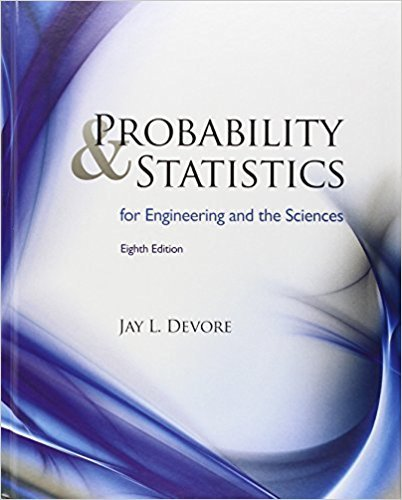×
×

# Solved: The value of Young’s modulus (GPa) was determinedISBN: 9780538733526 61

## Solution for problem 45E Chapter 1

Probability and Statistics for Engineering and the Sciences | 8th Edition

• Textbook Solutions
• 2901 Step-by-step solutions solved by professors and subject experts
• Get 24/7 help from StudySoup virtual teaching assistantsProbability and Statistics for Engineering and the Sciences | 8th Edition

4 5 0 353 Reviews
13
5
Problem 45E

The value of Young’s modulus (GPa) was determined for cast plates consisting of certain intermetallic substrates, resulting in the following sample observations (“Strength and Modulus of a Molybdenum-Coated Ti-25Al-10Nb-3U- 1Mo Intermetallic,” J. of Materials Engr. and Performance, 1997: 46–50):a. Calculate and the deviations from the mean.

b. Use the deviations calculated in part (a) to obtain the sample variance and the sample standard deviation.

c. Calculate s2 by using the computational formula for the numerator Sxx.

d. Subtract 100 from each observation to obtain a sample of transformed values. Now calculate the sample variance of these transformed values, and compare it to s2 for the original data.

Step-by-Step Solution:

Step 1 of 4</p>

a) We have to find the deviations from mean

The given values 116.4,  115.9,  114.6,  115.2,  115.8

Now Mean (=(116.4+115.9+114.6+115.2+115.8)/5

=577.9/5

= 115.58

The deviations from mean isStep 2 of 4</p>

b) We have to find sample variance and sample standard deviation from the deviations

Now sample variance (s)==(0.82)2+(0.32)2+(-0.98)2+(-0.38)2+(0.22)2/4

=1.928/4

=0.482

The sample standard deviation (s)=== 0.69

Hence sample variance=0.482 and the sample standard deviation=0.69

Step 3 of 4

Step 4 of 4

##### ISBN: 9780538733526

The full step-by-step solution to problem: 45E from chapter: 1 was answered by , our top Statistics solution expert on 08/08/17, 06:52AM. Since the solution to 45E from 1 chapter was answered, more than 312 students have viewed the full step-by-step answer. This textbook survival guide was created for the textbook: Probability and Statistics for Engineering and the Sciences , edition: 8. This full solution covers the following key subjects: sample, calculate, values, transformed, variance. This expansive textbook survival guide covers 16 chapters, and 1314 solutions. The answer to “The value of Young’s modulus (GPa) was determined for cast plates consisting of certain intermetallic substrates, resulting in the following sample observations (“Strength and Modulus of a Molybdenum-Coated Ti-25Al-10Nb-3U- 1Mo Intermetallic,” J. of Materials Engr. and Performance, 1997: 46–50): a. Calculate and the deviations from the mean.b. Use the deviations calculated in part (a) to obtain the sample variance and the sample standard deviation.c. Calculate s2 by using the computational formula for the numerator Sxx.d. Subtract 100 from each observation to obtain a sample of transformed values. Now calculate the sample variance of these transformed values, and compare it to s2 for the original data.” is broken down into a number of easy to follow steps, and 105 words. Probability and Statistics for Engineering and the Sciences was written by and is associated to the ISBN: 9780538733526.

Unlock Textbook Solution